# Algebraic Equations

### 3.3.4 Newton's Method

The following are all algebraic equations.

 x - 3 = 5 (1)

 sin 3x = y cos x (2)

 a x2 + b x + c = 0 (3)

• (1) is linear in x
• (2) is nonlinear in x because of its appearance in the trigonometric functions
• (2) is however linear in y
• (3) is nonlinear in x, but linear in a, b and c

### Analytical Solution

Only equations which are linear in their unknown have a guaranteed analytical solution:

 a x = b (4)

Or:

 a x - b =0 (5)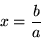However, consider: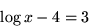(6)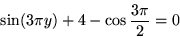(7)

### Solving a Single Algebraic Equation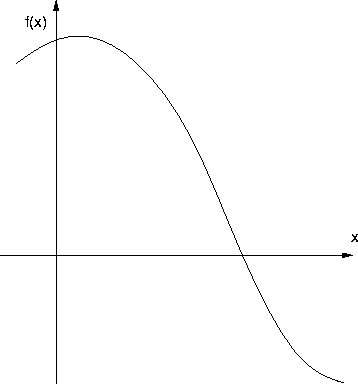Where is the solution ?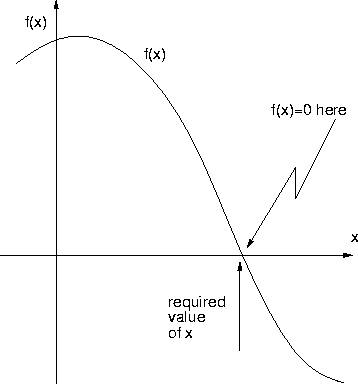It clearly lies on the x-axis at the point where the function f(x) value is zero.

Given an equation

f(x) = 0

find the value of x such that the function f(x) is zero to within some specified tolerance.

### Engineering Problems have Bounded Solutions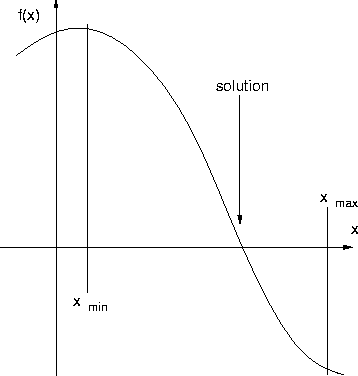Intrinsic:Operational: cooling water temperature, vessel pressure, etc

### 3.3.2 Simplest Method: Bisection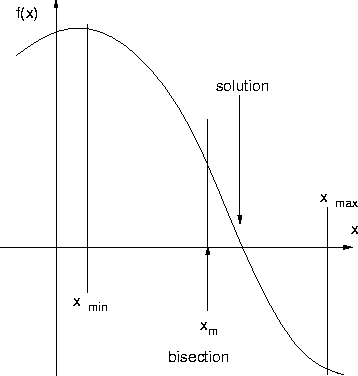First bisection gives a new, better x

Next discard redundant bound, here xmin

Replace it by xm which becomes new' xmin

Repeat

### Bisection Algorithm

1.
Specify bounds xmin and xmax
2.
Evaluate fl=f(xmin) and fu=f(xmax)
3.
Check that sign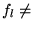sign fu. Abandon with error message if not so.
4.
Start iteration.
5.
Let xm = (xmin+xmax)/2
6.
Evaluate fm = f(xm)
7.
If sign fm = sign fl then make xmin = xm and fl=fm
8.
Else make xmax = xm and fu = fm
9.
If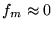then stop
10.
Repeat from step 4

Accuracy: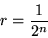### Method 2 - Secant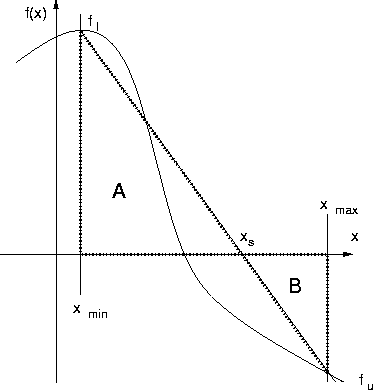Same algorithm as bisection, but a different way of choosing the new value.

### Secant Update

If f(x) is nearly linear between xmin and xmax, then a straight line between the point (xmin , fl) and (xmax , fu) will cross the x-axis at xs , close to the true solution of f(x)=0.

From similar triangles A and B: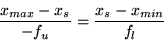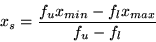New step 4:
1.
Let xm= (fu xmin - fl xmax)/(fu - fl)

### Method 3 - Newton-Raphson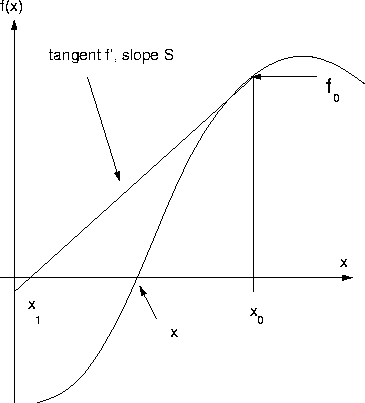Bisection uses only sign,

Secant uses value,

Newton also uses slope.

### Newton Update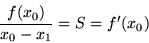Hence: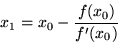Possible problems:
• Not bounded
• Needs reasonable starting guess
• Must differentiate

### Numerical Differentiation

By perturbing x by a small amountThen: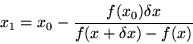<

NB, if we set:

### x0 = xmin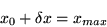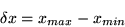...this gets back to the secant formula.

### A Method which Usually Doesn't Work

Methods involving rearrangement of:
f(x)=0

to the form:E.g. consider:

x3 +b x + c = 0

By alternative rearrangements: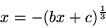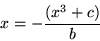Try with:
x3 - 0.01 x + 3 = 0

x3 -100 x - 1 =0

First equation can be solved by first rearrangement but not second. Second equation solves by second method but not first.

Sufficient condition for convergence is: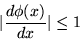at the solution

In general this class of repeated substitution method cannot be guaranteed to work. An exception is a situation where the physics of the system ensures that the above condition is always met. One example of this is in solving process recycle problems.

### A Chemical Process with Recycle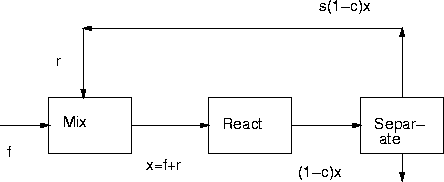Consider the recycle of a reactant.

If c is the fractional conversion in the reactor and s the fractional recovery of the recycled reactant in the separator, then the classic procedure of guessing a recycle stream' is equivalent to solving for x , the amount of reactant entering the reactor:

x = f + r

Here r=s(1-c)x) so:

x = f = s(1-c) x

Thus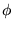is the RHS expression, i.e.: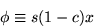And: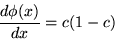Since c and s are both fractions, this must be less than unity.

Repeated substitution methods should be avoided unless it is certain that this condition is fulfilled. Even when it is, other methods are usually better.

Back to 3.3 Iterative Solution of Single Algebraic Equations

Back to Section 3 index.